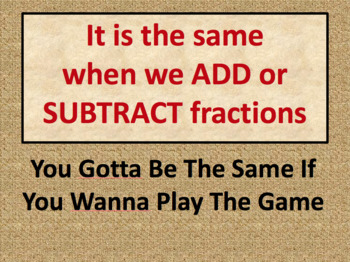# Add and Subtract Fractions with Unlike Denominators5th
Subjects
Standards
Formats Included
• PPTX
Pages
22 pages

### Description

This is a powerpoint presentation that introduces adding and subtracting fractions with unlike denominators. The presentation is animated and includes reasoning for finding common denominators, guided practice finding least common multiples, directions for a game, and student practice.
Total Pages
22 pages
N/A
Teaching Duration
N/A
Report this Resource to TpT
Reported resources will be reviewed by our team. Report this resource to let us know if this resource violates TpT’s content guidelines.

### Standards

to see state-specific standards (only available in the US).
Add and subtract fractions with unlike denominators (including mixed numbers) by replacing given fractions with equivalent fractions in such a way as to produce an equivalent sum or difference of fractions with like denominators. For example, 2/3 + 5/4 = 8/12 + 15/12 = 23/12. (In general, 𝘢/𝘣 + 𝘤/𝘥 = (𝘢𝘥 + 𝘣𝘤)/𝘣𝘥.)